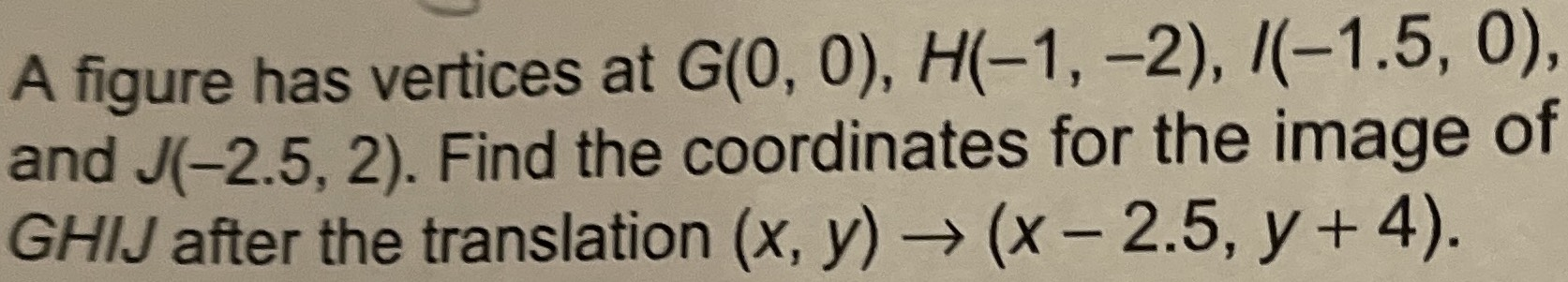### ¿Todavía tienes preguntas de matemáticas?

Pregunte a nuestros tutores expertos
Algebra
PreguntaA figure has vertices at $$G ( 0,0 ) , H ( - 1 , - 2 ) , I ( - 1.5,0 )$$ , and $$J ( - 2.5,2 )$$ . Find the coordinates for the image of $$G H I J$$ after the translation $$( x , y ) \rightarrow ( x - 2.5 , y + 4 )$$ .